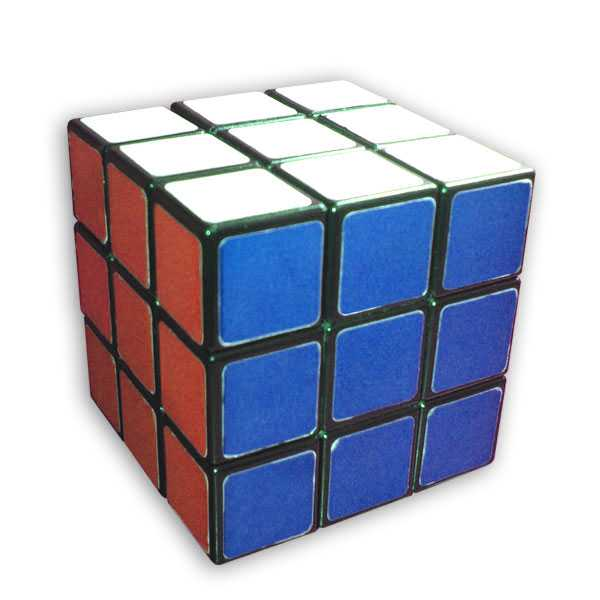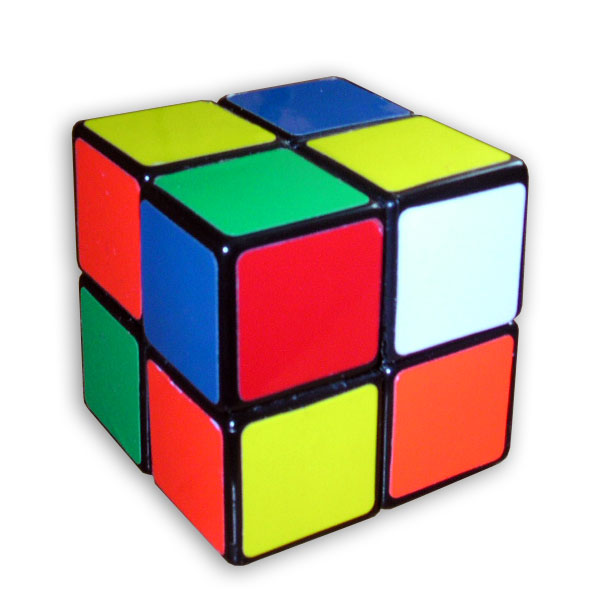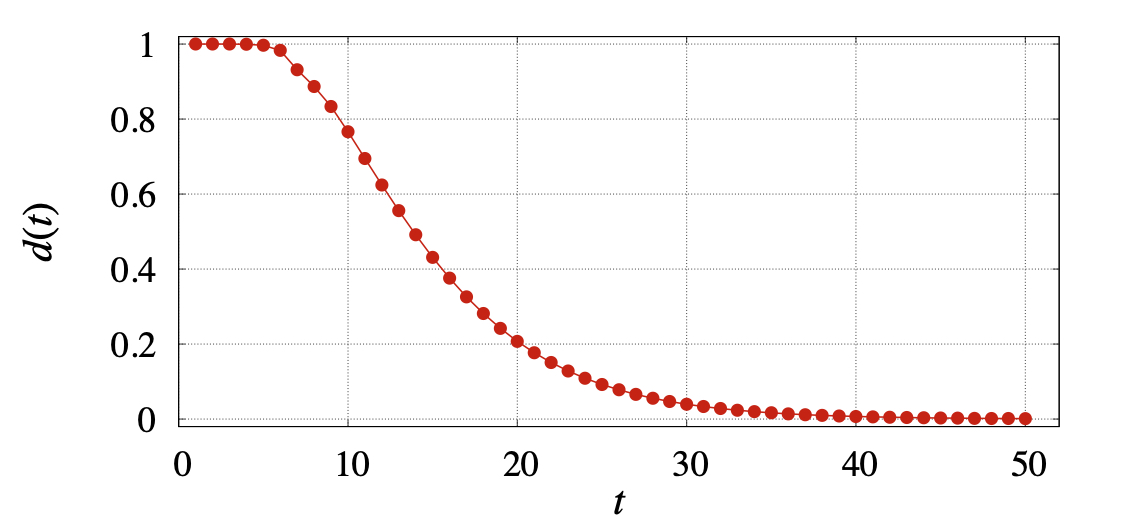Article sponsored by NewzEngine.com

Source: The Conversation (Au and NZ) – By Tim Garoni, Associate Professor, School of Mathematics, Monash University

Rubik’s Cube has been one of the world’s favourite puzzles for 40 years. Several different methods have been devised for solving it, as explained in countless books. Expert “speedcubers” can solve it in a matter of seconds.

In addition to such feats of astounding dexterity, there are many fascinating mathematical questions related to Rubik’s Cube. A move of the cube consists of rotating one of the six faces by either 90, 180, or 270 degrees. A staggering 43,252,003,274,489,856,000 possible states can be obtained by applying sequences of moves to the solved state.

Read more: How to solve a Rubik’s cube in five seconds

Despite this complexity, it was shown in 2010 that Rubik’s Cube can always be solved in 20 moves or fewer, regardless of the initial state. This number is referred to as “God’s number”, as all known solution methods used by humans typically use significantly more moves than this optimal value.Rubik’s Cube in the solved state. Mike Gonzalez (TheCoffee)

But what about the opposite question: how many moves are required to scramble a solved cube? At first glance, this sounds like a much easier question than computing God’s number. After all, unlike solving a cube, scrambling one takes no skill whatsoever.

Similar questions have been answered successfully for card shuffling. A famous example is the 1990 study of the “riffle shuffle” by mathematicians Dave Bayer and Perci Diaconis. A deck of cards is defined as “mixed” if its ordering is random, with each possible order having the same probability of appearing. Bayer and Diaconis showed that seven riffle shuffles are necessary and sufficient to approximately mix a standard deck of playing cards.

Last year, mathematicians published a similar study of the 15 puzzle, which consists of a 4×4 square filled with 15 sliding tiles and one empty space.

## What does it mean for a cube to be scrambled?

A typical person trying to scramble a Rubik’s Cube would repeatedly perform random moves on it. The resulting random sequence of states is a special case of what mathematicians call a Markov chain. The key property is that given the current state, the probability of what the next state will be does not depend on any of the previous states.

Applying the theory of Markov chains to cube scrambling, it follows that as the number of random moves increases, the probability of being in any particular one of the possible states becomes closer and closer to 1/43,252,003,274,489,856,000. Mathematicians call this a “uniform probability distribution”, as each possible state occurs with the same probability.

After any given number of random moves, the state of the cube will be random, but its probability distribution will not be exactly uniform; some states will be more likely to occur than others.

Let d(t) describe how much the probability distribution after t random moves differs from the uniform probability distribution. As the number of random moves (t) increases, the value of d(t) will decrease. The cube being scrambled corresponds to d(t) being small.

## Markov-chain Monte Carlo

In the theory of Markov chains, this decrease in d(t) is called “mixing”. Besides card shuffling and puzzle scrambling, the theory of Markov chain mixing also has very serious practical applications. One of the most important computational tools in modern science and engineering is the Monte Carlo method. This method, like the famous casino after which it is named, relies fundamentally on chance. In essence, it attempts to approximately solve hard mathematical problems using multiple random guesses.

In practice, Markov chains are often used to produce these random states. To understand the accuracy of these Markov-chain Monte Carlo methods, the key task is to estimate how quickly d(t) decreases as t increases.

## The pocket cubePocket cube in a scrambled state. Mike Gonzalez (TheCoffee)

Studying the scrambling problem for the standard 3x3x3 Rubik’s Cube is currently a fascinating unsolved challenge. However, it becomes quite manageable if we turn our attention to a smaller 2x2x2 version, called the pocket cube.

In this cube, the edge and centre pieces are absent and only the corner pieces remain. The pocket cube has only 3,674,160 possible states, and its God’s number is only 11.

In the graph below, we plot d(t) for the pocket cube. After 11 moves, d(t) is still very large, at 0.695. The first value of t that yields a d(t) value below 0.25 (often called “the mixing time” in Markov chain theory) is 19. After 25 moves d(t) is 0.092; after 50 moves it is 0.0012; and after 100 moves it is 0.00000017.Distance of the pocket cube distribution from uniform after t moves. Eric Zhou

So how many moves should you use to fully scramble a pocket cube? The answer depends on how small you would like d(t) to be. However, it is certainly true that God’s number of moves is insufficient. As a bare minimum, one should not use fewer than 19 moves. Further details, including code to compute d(t), are available here.

And of course, once you’ve scrambled your cube, all that’s left to do is solve it again.

Read more: Your guide to solving the next online viral maths problem

ref. How hard is it to scramble Rubik’s Cube? – https://theconversation.com/how-hard-is-it-to-scramble-rubiks-cube-129916